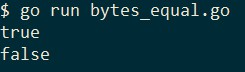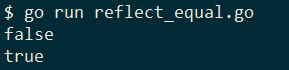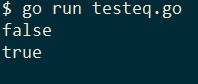## golang slice is determined whether the two are equal

In golang we can easily pass `==`to determine whether two arrays (array) are equal, but unfortunately did not slice the relevant operator, when two slice need to determine whether we can find another shortcut to the equal.

## slice the definition of equal

We chose the most common demand, that is, when the same two slice type and length, and equal under the target value is equal to, for example:

``````a := []int{1, 2, 3}
b := []int{1, 2, 3}
c := []int{1, 2}
d := []int{1, 3, 2}``````

The above code `a`and `b`are equal, `c`because the length and `a`different it is not equal, `d`because the order of elements and `a`different so do not equal.

## Analyzing two [] byte are equal

Why should we separate the [] byte list out of it?

Because the standard library provides optimized ICP, we no longer need to make the wheel:

``````package main

import (
"bytes"
"fmt"
)

func main() {
a := []byte{0, 1, 3, 2}
b := []byte{0, 1, 3, 2}
c := []byte{1, 1, 3, 2}

fmt.Println(bytes.Equal(a, b))
fmt.Println(bytes.Equal(a, c))
}``````

Results are as follows:## Determining whether the same slice reflect the use of

When not determining the type [] byte of Slice, we can also aid `reflect.DeepEqual`, which is used to compare two objects comprises depth if they contain internal elements are equal:

``func DeepEqual(x, y interface{}) bool``

DeepEqual reports whether x and y are “deeply equal,” defined as follows. Two values of identical type are deeply equal if one of the following cases applies. Values of distinct types are never deeply equal.
...
Slice values are deeply equal when all of the following are true: they are both nil or both non-nil, they have the same length, and either they point to the same initial entry of the same underlying array (that is, &x == &y) or their corresponding elements (up to length) are deeply equal. Note that a non-nil empty slice and a nil slice (for example, []byte{} and []byte(nil)) are not deeply equal.

Meaning of this passage is not difficult to understand, how to determine the slice equal principles and we discussed at the beginning of this article is the same, except that it means "black magic" a little run-time.

Look at an example:

``````package main

import (
"fmt"
"reflect"
)

func main() {
a := []int{1, 2, 3, 4}
b := []int{1, 3, 2, 4}
c := []int{1, 2, 3, 4}
fmt.Println(reflect.DeepEqual(a, b))
fmt.Println(reflect.DeepEqual(a, c))
}``````## Handwriting judgment

In golang in use reflect the cost of performance usually takes, if we determine the type of slice, then achieve their own slice of relatively equal judgment is not so much trouble:

``````func testEq(a, b []int) bool {
// If one is nil, the other must also be nil.
if (a == nil) != (b == nil) {
return false;
}

if len(a) != len(b) {
return false
}

for i := range a {
if a[i] != b[i] {
return false
}
}

return true
}``````

Test code:

``````package main

import "fmt"

func main() {
a := []int{1, 2, 3, 4}
b := []int{1, 3, 2, 4}
c := []int{1, 2, 3, 4}
fmt.Println(testEq(a, b))
fmt.Println(testEq(a, c))
}``````

operation result:Of course, we do have an obvious drawbacks, as we have many types of slice we have to write different versions `testEq`, but they are the only difference with only slice type.

But wait until go2 generic can be used, such defects would be gone, and now what we need is to make a trade-off between complexity and operational performance of the code.

##### reference

Checking the equality of two slices

### Guess you like

Origin www.cnblogs.com/apocelipes/p/11116725.html
Recommended
Ranking
Daily